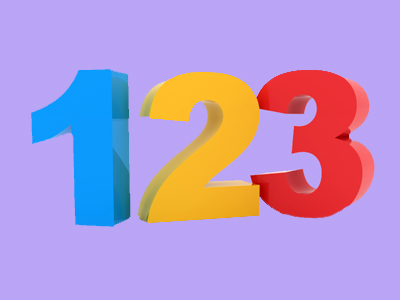In the number 123 there are 3 ones, 2 tens and 1 hundred.

# Year 2 Numbers - Place Value and Comparing

This quiz addresses the requirements of the National Curriculum KS1 Maths and Numeracy for children aged 6 and 7 in year 2. Specifically this quiz is aimed at the section dealing with comparing numbers to at least 100.

Understanding the place value of digits within numbers helps to order and compare numbers up to one hundred. Children in Year 2 should begin to use this knowledge to help them order numbers up to 100 from smallest to largest, place numbers on a number line and compare numbers, knowing which have smaller or larger values than others.

Question 1
What is the value of the 7 in 27?
7 ones
7 tens
7 hundreds
7 thousands
The last digit in a whole number represents ones
Question 2
Which numbers come immediately before and after 79?
78 and 80
77 and 78
69 and 89
67 and 76
One less than 79 is 78 and one more is 80
Question 3
What is the value of the 5 in 57?
5 hundreds
5 ones
5 tens
5 thousands
This column in a number represents the tens
Question 4
Put these numbers in order from highest to lowest:
45, 14, 41, 54, 15
54, 45, 41, 15, 14
45, 54, 14, 41, 15
14, 15, 41, 45, 54
15, 45, 54, 14, 41
54 has the highest value, 14 has the lowest value
Question 5
What is 67 in words?
Six and seven
Sixty-seven
Sixteen
Seventy
The two words have a hyphen between them
Question 6
Which number is the smallest?
16, 61, 66, 11
61
16
11
66
11 is smaller than the other numbers
Question 7
Which numbers are missing?
__, 46, __, __
40, 50, 60
46, 47, 48
55, 56, 57
45, 47, 48
Thinking about the numbers on a number line might be useful here
Question 8
Put these numbers in order from lowest to highest:
23, 33, 32, 12, 13
32, 33, 23, 12, 13
33, 32, 23, 13, 12
32, 33, 13, 23, 12
12, 13, 23, 32, 33
The lowest number is 12 and the highest is 33
Question 9
Which is the largest number?
37, 73, 13, 17
73
37
13
17
73 has 7 tens and 3 ones
Question 10
Which numbers are missing?
23, 24, __, 26, __, __
27, 28, 29
24, 25, 26
25, 27, 28
28, 38, 48
Counting up aloud might help here